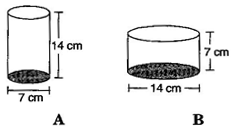# Diameter of cylinder A is 7 cm and the height is 14 cm. Diameter of cylinder B is 14 cm and height is 7 cm. Without doing any calculations can you suggest whose volume is greater? Verify it by finding the volume of both the cylinders. Check whether the cylinder with greater volume also has greater surface area.Solution

Cylinder A:

Radius of cylinder A = 7/2 cm

Height of cylinder A = 14 cm

Volume of cylinder A = ∏.r2h

= 22/7 x 7/2 x 7/2 x 14

= 539 cm3

Total Surface area of cylinder A = 2.∏.r(h + r)

= 2 x 22/7 x 7/2(14 + 7/2)

= 385 cm2

Cylinder B:

Radius of cylinder B = 14/2 or 7 cm

Height of cylinder B = 7 cm

Volume of cylinder B = ∏.r2h

= 22/7 x 7 x 7 x 7

= 1078 cm3

Total Surface area of cylinder B = 2.∏.r(h + r)

= 2 x 22/7 x 7(7 + 7)

= 616 cm2

We get to know that see that the Volume of cylinder B is twice that of cylinder A.

Hence, we conclude that the Volume of cylinder B is greater than the volume of cylinder A.

Total surface area of cylinder B is greater.